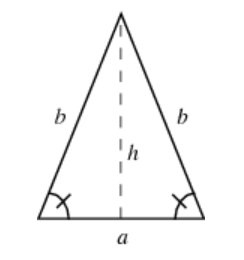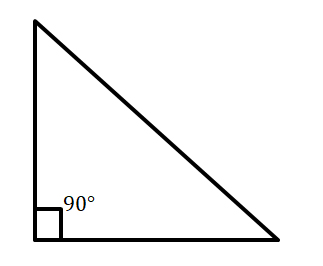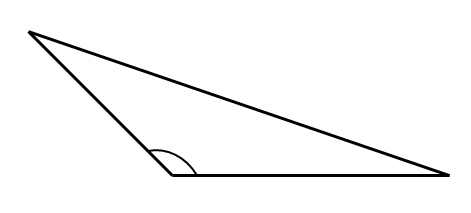Triangles - Geometry
28-06-2020    106 times### Triangles

If a polygon designed with three lines or line segments and three vertexes, then this polygon is known as triangles. The sum of the internal angles of any triangles is 180°.

### Types of triangle

Triangles are shapes with three sides. There are different names for the types of triangles. A triangle’s types depend on the length of its sides and the size of its angles.

There are three types of triangle based on the length of the sides:

1. Equilateral: An equilateral triangle has three equal length sides and three equal inside angles. Each of the angles measures 60°2. Isosceles: An isosceles triangle has two equal length sides. It also has two inside angles that are equal size. Other angle can be acute(less than 90°), a right angle(equals to 90°), or obtuse (more than 90°).3. Scalene: A scalene triangle has no equal length sides. It also has no equal inside angles. These triangles can be acute (all angles less than 90°)A triangle’s name also depends on the size of its inside angles1. Acute Triangles: If all the angles are less than 90°2. Right Angled Triangles: A right angled triangles has one inside angle that is a right angle (90°). These triangles can be isosceles or scalene. This is a right-angled scalene triangle because no sides are the same length. The sides opposite the right angle are called the hypotenuse.3. Obtuse Triangles: if one angle is more than 90deg;